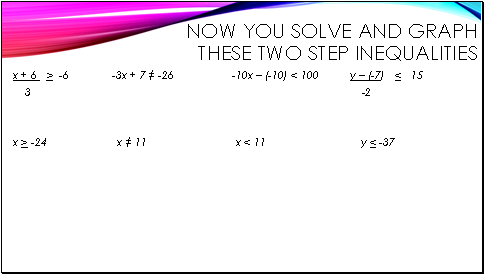# Maths InequalitiesPage 2

#### WATCH ALL SLIDES

3x < 9 still not done

÷3 ÷3 opposite of multiply is divide

x < 3 solution now we can graph

x + 6 > -6 given

3

3(x + 6) > (-6) 3 opposite of divide is multiply

3

x + 6 > -18 still not done

x + 6 – 6 > -18 – 6 now opposite of addition is subtraction

x > -24 now graph the solution

-5y – (-15) < -30 given

-5y + 15 < -30 change subtraction to addition

-5y + 15 – 15 < -30 – 15 opposite of addition is subtraction

-5y < -45 not done yet

-5y ÷ -5 < -45 ÷ -5 opposite of multiply is divide

y > 9 have to flip sign and now we have the solution to graph

Slide 10Now you solve and Graph these two step inequalities

x + 6 > -6 -3x + 7 ≠ -26 -10x – (-10) < 100 y – (-7) < 15

3 -2

x > -24 x ≠ 11 x < 11 y < -37

Go to page:
1  2